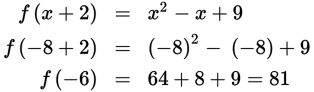# SAT Math Multiple Choice Question 923: Answer and Explanation

### Test Information

Question: 923

7. If f(x + 2) = x2x + 9, then what is the value of f(–6)?

• A. –47
• B. 29
• C. 65
• D. 81

Explanation:

D

Difficulty: Hard

Category: Passport to Advanced Math / Functions

Strategic Advice: In a question like this, where a shifted function is given (x + 2) but a value for the original function is requested, try to write the input (the number inside the parentheses) in terms of the shift.

Getting to the Answer: Start by writing -6 in terms of x + 2 (in other words, some number plus 2): -6 can be written as -8 + 2, so x is -8. Now, substitute this value into the function: